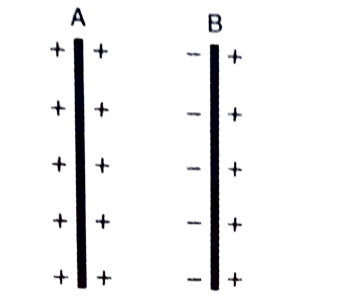# Principle of a capacitor – step by step

## Principle of a capacitor

The principle of a capacitor is based on two physical events. These are as follows:

1 ) The capacitance of a conductor increases by a small amount when another uncharged conductor is placed near it.
2 ) The capacitance of a conductor increases greatly when an earth-connected conductor is placed near it.

A capacitor is a device for storing a large quantity of electric charge.

A conductor can store a small amount of charge and hence it possesses a small capacitance. To store a large amount of charge, two (or more) conductors are arranged to form a capacitor. Thus, a capacitor possesses a large capacitance to store charge, and that too in a small space.

Detailed discussion:

### The capacitance of a conductor increases by a small amount when another uncharged conductor is placed near it.The capacitance of a conductor increases by a small amount when another uncharged conductor is placed near it.
• consider an insulated metal plate A. Suppose that it is given a positive charge, till its potential becomes maximum.
• At this point, the plate won’t hold any more charge over it. If any more charge is given to the metal plate A, it will leak to the surroundings.
• Now, let’s place another metallic plate B near plate A.
• due to induction, the negative charge will be induced on the nearer face of plate B and the positive charge will be induced on the farther face of plate B.
• The potential of plate A gets lowered due to the induced negative charge on plate B and a bit raised due to the induced positive charge on plate B.
• Since the metal plates have a finite thickness, the induced negative charge is nearer to plate A than the induced positive charge on plate B is.
• therefore, on the whole, the potential of plate A gets lowered by a very small amount.
• In order to make the potential of the metal plate A again the same, an additional small amount of charge has to be given to plate A.
• It means the capacitance of a conductor increases by a small amount when another uncharged conductor is placed near it.